Students can download Maths Chapter 3 Algebra Ex 3.6 Questions and Answers, Notes, Samacheer Kalvi 10th Maths Guide Pdf helps you to revise the complete Tamilnadu State Board New Syllabus, helps students complete homework assignments and to score high marks in board exams.

## Tamilnadu Samacheer Kalvi 10th Maths Solutions Chapter 3 Algebra Ex 3.6

Question 1.
Simplify
(i) $$\frac{x(x+1)}{x-2}+\frac{x(1-x)}{x-2}$$
$$\frac{x(x+1)}{x-2}+\frac{x(1-x)}{x-2}$$ = $$\frac{x(x+1)+x(1-x)}{x-2}$$
= $$\frac{x^{2}+x+x-x^{2}}{x-2}$$
= $$\frac { 2x }{ x-2 }$$

(ii) $$\frac { x+2 }{ x+3 }$$ + $$\frac { x-1 }{ x-2 }$$
$$\frac { x+2 }{ x+3 }$$ + $$\frac { x-1 }{ x-2 }$$ = $$\frac{(x+2)(x-2)+(x-1)(x+3)}{(x+3)(x-2)}$$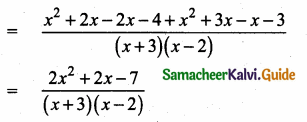(iii) $$\frac{x^{3}}{x-y}+\frac{y^{3}}{y-x}$$ = $$\frac{x^{3}}{x-y}+\frac{y^{3}}{-1(x-y)}$$
= $$\frac{x^{3}}{x-y}-\frac{y^{3}}{x-y}$$
= $$\frac{x^{3}-y^{3}}{x-y}$$ (using a3 – b3 = (a – b) (a2 + ab + b2))
= $$\frac{(x-y)\left(x^{2}+x y+y^{2}\right)}{x-y}$$
= x2 + xy + y2

Question 2.
Simplify
(i) $$\frac{(2 x+1)(x-2)}{x-4}$$ – $$\frac{\left(2 x^{2}-5 x+2\right)}{x-4}$$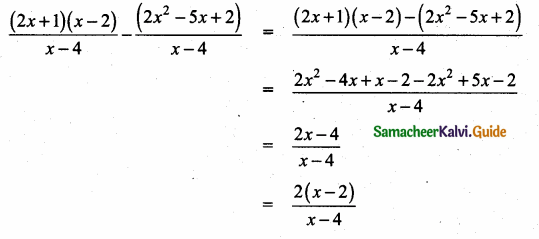(ii) $$\frac{4 x}{x^{2}-1}-\frac{x+1}{x-1}$$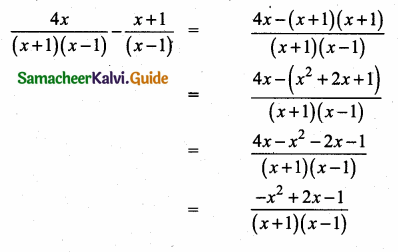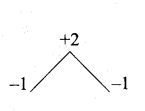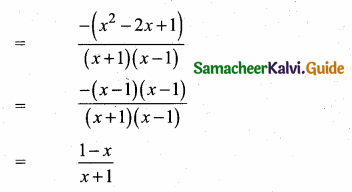Question 3.
Subtract $$\frac{1}{x^{2}+2}$$ from $$\frac{2 x^{3}+x^{2}+3}{\left(x^{2}+2\right)^{2}}$$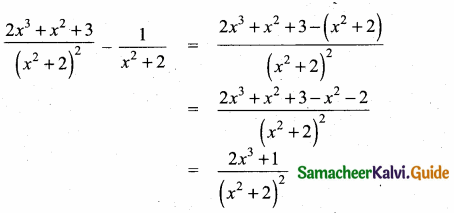Question 4.
Which rational expression should be subtracted from $$\frac{x^{2}+6 x+8}{x^{3}+8}$$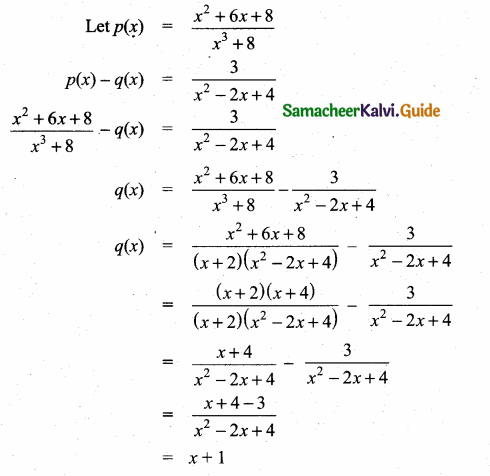Question 5.
If A = $$\frac{2x+1}{2 x-1}$$, B = $$\frac{2x-1}{2x+1}$$ find $$\frac{1}{A-B}$$ – $$\frac{2 \mathbf{B}}{\mathbf{A}^{2}-\mathbf{B}^{2}}$$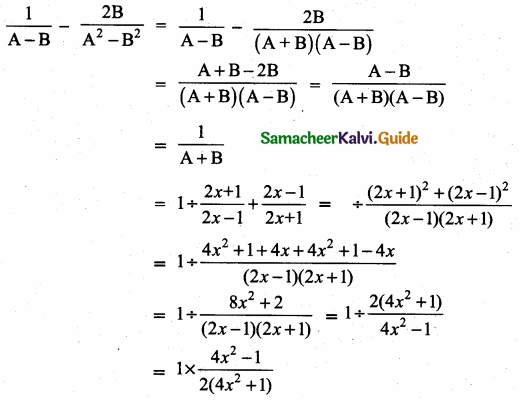Question 6.
If A = $$\frac { x }{ x+1 }$$ B = $$\frac { 1 }{ x+1 }$$ prove that $$\frac{(\mathbf{A}+\mathbf{B})^{2}+(\mathbf{A}-\mathbf{B})^{2}}{\mathbf{A}+\mathbf{B}}=\frac{2\left(x^{2}+1\right)}{x(x+1)^{2}}$$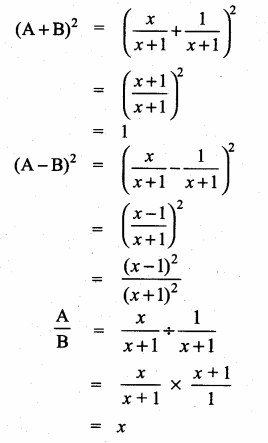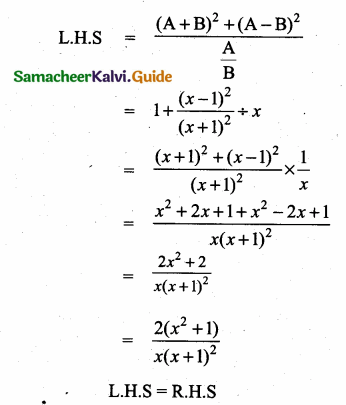Question 7.
Pari needs 4 hours to complete a work. His friend Yuvan needs 6 hours to complete the same work. How long will it take to complete if they work together?
Solution:
Pari: time required to complete the work = 4 hrs.
∴ In 1 hr. he will complete = $$\frac{1}{4}$$ of the work.
= $$\frac{1}{4}$$ w.
Yuvan: Time required to complete the work = 6 hrs.
∴ In 1 hr. he will complete the $$\frac{1}{6}$$ of the work
= $$\frac{1}{6}$$ w
Working together, in 1 hr. they will complete $$\frac{w}{4}+\frac{w}{6}$$ of the work.
= $$\frac{6 w+4 w}{24}=\frac{5}{12} w$$
∴ To complete the total work time taken
= $$\frac{w}{\frac{5}{12} w}=\frac{12}{5}$$ = 2.4 hrs. [∵ (4) hrs = 4 × 60 = 24 min]
= 2 hrs 24 minutes.Question 8.
Iniya bought 50 kg of fruits consisting of apples and bananas. She paid twice as much per kg for the apple as she did for the banana. If Iniya bought ? 1800 worth of apples and ₹ 600 worth bananas, then how many kgs of each fruit did she buy?
Let the weight of applies be a kg.
Let the weight of bananas be b kg.
a + b = 50
ax = ₹ 1800 ………….. (1)
by = ₹ 600 ………… (2)
x = 2y …………… (3)
Use (3) in (1) ⇒ a(2y) = 1800
y = $$\frac{900}{a}$$ ………….. (4)
Use (4) in (2) ⇒ $$b\frac{900}{a}$$ = 600
∵ 3b = 2a …………….. (5)
∵ a + b = 50
a + $$\frac{2 a}{3}$$ = 50 ⇒ $$\frac{5 a}{3}$$ = 50
⇒ a = 50 × $$\frac{3}{5}$$
= 30
∴ b = 20
∴ Iniya bought 30 kg of applies and 20 kg of bananas
= 30
.’. b = 20.
.’. Iniya bought 30 kg of applies and 20 kg of bananas.